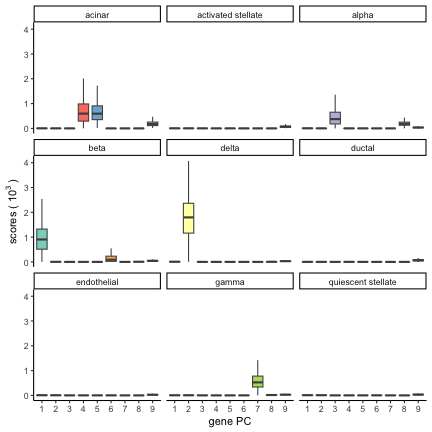# Explore multivariate data with epca

### 2023-07-10

This vignette walks you through how you could easily use epca to explore your data.

## Quick Start

Using sca and sma is simple. The only required input are the data matrix x and k–the number of sparse PCs (or rank of matrix decomposition).

To illustrate, we simulated a $$300 \times 50$$ example rank-5 data matrix x with some additive Gaussian noise (the steps to generate this matrix is skipped; look for the source code of this vignette for details).

sca(x, k = 5)

## Call:sca(x = x, k = 5)
##
##
## Cumulative proportion of variance explained (CPVE):
##                      CPVE
## First component:    0.097
## First 2 components: 0.194
## First 3 components: 0.288
## First 4 components: 0.380
## First 5 components: 0.410

sma(x, k = 5)

## Call: sma(x = x, k = 5)
##
##
## Num. non-zero z's:  178 161 178 168 215
## Num. non-zero y's:  18 27 26 23 24
## Abs. sum z's (L1-norm):  9.132387
## Abs. sum y's (L1-norm):  3.274192


## Example 1: pitprops data

In this example, we apply sca to the pitprops data set. This data is a Gram matrix (i.e., covariance matrix). For this, we just need to set is.Cov = TRUE. We look for 6 sparse PCs and set the sparsity parameter gamma = 6. Here, the sparsity parameter controls the L1 norm of the returned PC loadings. The default of gamma (if absent) is sqrt(p * k), where p is the number of original variables. This default of gamma is usually well sufficiently large.

data("pitprops", package = "epca")
s.sca <- sca(pitprops, k = 6, gamma = 6)


There are two ways to inspect the sparse PC loadings. The first way is to directly extract the loadings component in the output object: s.sca$loadings. The second option is to call the print generic function with the verbose = TRUE option. It prints the original variables with non-zero loadings for each PC sequentially. print(s.sca, verbose = TRUE)  ## Call:sca(x = pitprops, k = 6, gamma = 6) ## ## ## Num. non-zero loadings': 6 2 4 4 3 2 ## Abs. sum loadings' (L1-norm): 1.183285 ## Cumulative proportion of variance explained (CPVE): ## CPVE ## First component: 0.265 ## First 2 components: 0.396 ## First 3 components: 0.538 ## First 4 components: 0.663 ## First 5 components: 0.768 ## First 6 components: 0.840 ## ## Component 1 : ## ## feature loadings ## 1 topdiam 0.299 ## 2 length 0.312 ## 3 ovensg -0.189 ## 4 bowmax 0.047 ## 5 bowdist 0.2 ## 6 whorls 0.136 ## ## Component 2 : ## ## feature loadings ## 1 moist 0.519 ## 2 testsg 0.493 ## ## Component 3 : ## ## feature loadings ## 1 ovensg 0.092 ## 2 ringtop 0.497 ## 3 ringbut 0.323 ## 4 bowmax -0.162 ## ## Component 4 : ## ## feature loadings ## 1 length 0.006 ## 2 bowmax -0.227 ## 3 whorls -0.037 ## 4 diaknot 0.619 ## ## Component 5 : ## ## feature loadings ## 1 ovensg -0.253 ## 2 bowmax -0.058 ## 3 knots 0.638 ## ## Component 6 : ## ## feature loadings ## 1 whorls -0.175 ## 2 clear 0.717  ## Example 2: single-cell RNA-seq data This example shows a large-scale application of sparse PCA to a single-cell RNA-seq data. For this example, we use the human/mouse pancreas single-cell RNA-seq data from Baron et al. (2017). Fe used the single-cell RNA-seq data with the scRNAseq package. We removed the genes that do not have any variation across samples (i.e., zero standard deviation) and the cell types that contain fewer than 100 cells. This resulted in a sparse data matrix pancreas of 17499 genes (rows) and 8451 cells (columns) across nine cell types. dat <- BaronPancreasData("human") gene.select <- !!apply(counts(dat), 1, sd) label.select <- colData(dat) %>% data.frame() %>% dplyr::count(label) %>% filter(n > 100) dat1 <- dat[gene.select, colData(dat)$label %in% label.select$label]  For SCA, we use the expression count matrix (count) as the input, where count[i,j] is the expression level of gene j in cell i, with 10.8% being non-zero. count <- counts(dat1)  The dataset contains labels for each cell. label <- setNames(factor(dat1$label), colnames(dat1))


Next, We applied sca to the transpose of count to find k = 9 sparse gene PCs. Aiming for a small number of genes (i.e., non-zero loadings) in individual PCs, we set the sparsity parameter to gamma = log(pk), which is approximately 12.

scar <- sca(t(count), k = 9, gamma = 12, center = F, scale = F, epsilon = 0.001)


We can exam the number of original genes included by each gene PC.

n.gene <- apply(!!scar$loadings, 2, sum) n.gene  ## PC1 PC2 PC3 PC4 PC5 PC6 PC7 PC8 PC9 ## 1 1 1 8 15 1 1 3 61  Each gene PC uses a handful of original genes. We can plot the component scores of the nine PCs, with dplyr and ggplot2 packages. Each panel displays one of nine cell types with the names of cell types and the number of cells reported on the top strips. For each cell type, a box depicts the component scores for nine sparse gene PCs. scar$scores %>%
reshape2::melt(varnames = c("cell", "PC"), value.name = "scores") %>%
mutate(PC = factor(PC), label = label[cell]) %>%
ggplot(aes(PC, scores/1000, fill = PC)) + geom_boxplot(color = "grey30", outlier.shape = NA,
show.legend = FALSE) + labs(x = "gene PC", y = bquote("scores (" ~ 10^3 ~ ")")) +
scale_x_discrete(labels = 1:9) + facet_wrap(~label, nrow = 3) + scale_fill_brewer(palette = "Set3") +
theme_classic()We observed that most of the gene PCs consist of one or a handful of genes, yet the component scores showed that these PCs distinguish different cell types effectively . For example, the PC 2 consists of only one gene (named SST), and the expression of the gene marks the “delta” cells among others. This result highlights power of scRNA-seq in capture cell-type specific information and suggests the applicability of our methods to biological data.